# CAT Prep 2022 | CAT Previous Year Question Papers With Solution | CAT 2022 Student Review | PaGaLGuY

Join this group to gain free access to CAT question papers as well as possible solutions. Get expert advice from our alumni network on the best ways to solve problems in verbal and reading comprehension (VARC), data interpretation and logical reasoning (DI & LR), quantitative ability (QA), and more.7 Likes

#### CAT 2021 Quants Question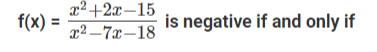1. -5 < x < -2 or 3 < x < 9
2. -2 < x < 3 or x > 9
3. x < -5 or 3 < x < 9
4. x < -5 or -2 < x < 3
2 LikesKeeping these roots on the number line and checking for the range where f(x) becomes negative.
For the numbers below -5, f(x) is positive.
For the numbers between -5 and -2, the value f(x) is negative.
For the numbers between -2 and 3, the value of f(x) is positive.
For the numbers between 3 and 9, the value of f(x) is negative.
For the numbers above 9, the value of f(x) is positive.
Hence the range should be -5 < x < -2 and 3 < x < 9.##### Hence, the answer is ‘-5 < x < -2 or 3 < x < 9’

Choice A is the correct answer.

1 Like

CAT 2021 Quant Question

The number of integers n that satisfy the inequalities |n - 60| < |n - 100| < |n - 20| is

1. 21
2. 18
3. 20
4. 19
1 Like

|n - 60| < |n - 100| < |n - 20|
To solve this question, we need to know the basic concept that ‘if x and y are two points on the number line, then the distance between these two points can be represented by |x - y| or |y - x|.’
Let’s take the first part of the inequality
|n - 60| < |n - 100|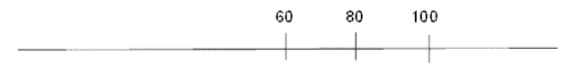This inequality holds good for the values of n below 80. So this is the turning point.
Hence ‘n’ should be less than 80.
Now Let’s check for the later part of the inequality.
|n - 100| < |n - 20|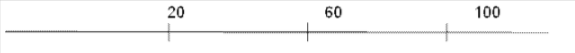This inequality holds good for the values of n above 60. So, 60 is the turning point here.
Hence ‘n’ should be greater than 60.
Therefore, the values of ‘n’ range from 61 to 79.
So, the total possible integers that satisfy this inequality are 19.

The question is " The number of integers n that satisfy the inequalities |n - 60| < |n - 100| < |n - 20| is "

##### Hence, the answer is ‘19’

Choice 4 is the correct answer.

Hello all, I wanted to apply to IMDR, but some of the reviews I read from alumni were confusing. I do want to be close to home, but I also know that your business school needs to be recommended because that’s what companies look for when hiring a new manager.

1 Like

I am a fresher with a BCom background; which of these colleges should be in my top 2 options?

• PIBM
• SSIB
• BIIB
• IMDR

0 voters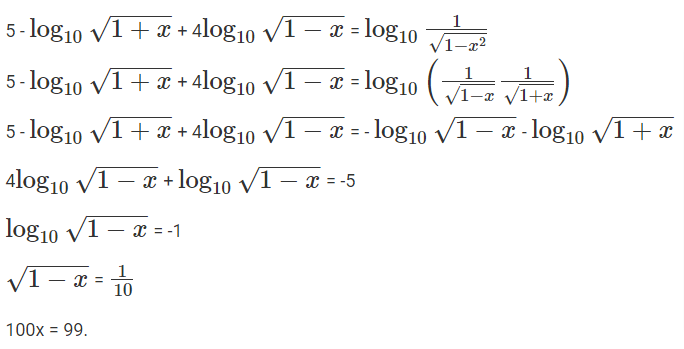Therefore the answer should be 99.

Let’s consider Apple, orange, mango to be x, y, z respectively.
Let’s take the first two baskets and solve from there.
2x + 4y + 6z = x + 4y + 8z
x = 2z.
Now, let’s take any other two different baskets and try to find the number of oranges in terms of the number of mangoes.
2x + 4y + 6z = 8y + 7z
2(2z) + 4y + 6z = 8y + 7z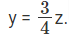We need to find the value of one basket in terms of mangoes. So substitute x and y values in any one of the equations.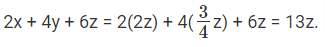13 mangoes worth of fruits is there in every basket.

Choice B is the correct answer.

1 Like

CAT 2021 Quant Question

Identical chocolate pieces are sold in boxes of two sizes, small and large. The large box is sold for twice the price of the small box. If the selling price per gram of chocolate in the large box is 12% less than that in the small box, then the percentage by which the weight of chocolate in the large box exceeds that in the small box is nearest to

A. 127
B. 135
C. 124
D. 144

CAT 2021 Quant Question

If the area of a regular hexagon is equal to the area of an equilateral triangle of side 12 cm, then the length, in cm, of each side of the hexagon is

A. 4 √6
B. 6 √6
C. 2 √6
D. √6

Let’s consider the selling prices of small and large chocolate boxes be ‘x’ and ‘2x’ respectively.
Let’s also consider the weights of the small and large chocolate boxes to be 100(to make it easy) and y respectively.
Given that, the selling price per gram of chocolate in the large box is 12% less than that in the small box.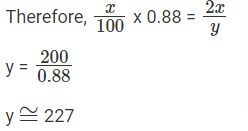Hence, the weight of chocolate in a large box exceeds 127% of that of a small box.
Choice A is the correct answer.

Given that, the area of a regular hexagon is equal to the area of an equilateral triangle of side 12cm.
Let’s consider ‘a’ be the side of the regular hexagon.a2 = 24
a = 2√6
Hence, the answer is 2 √6
Choice C is the correct answer.

CAT 2021 Quant Question

Onion is sold for 5 consecutive months at the rate of Rs 10, 20, 25, 25, and 50 per kg, respectively. A family spends a fixed amount of money on onion for each of the first three months, and then spends half that amount on onion for each of the next two months. The average expense for onion, in rupees per kg, for the family over these 5 months is closest to

A. 18
B. 26
C. 16
D. 20

Given that onion is sold for 5 consecutive months at the rate of Rs 10, 20, 25, 25, and 50 per kg respectively.

Let’s consider the amount spend on onions in these five months to be 100, 100, 100, 50, 50.

Hence, the number of kgs in these five months should be 10, 5, 4, 2, 1 respectively.

The average expense on onions per kg over these five months is given by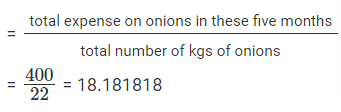Therefore, the closest average would be 18.

Choice A is the correct answer.

CAT 2021 Quant Question

Amal purchases some pens at ₹ 8 each. To sell these, he hires an employee at a fixed wage. He sells 100 of these pens at ₹ 12 each. If the remaining pens are sold at ₹ 11 each, then he makes a net profit of ₹ 300, while he makes a net loss of ₹ 300 if the remaining pens are sold at ₹ 9 each. The wage of the employee, in INR, is

Let’s consider the total number of pens to be
(100 +x)
Let’s consider the fixed-wage of the labour to be ‘w’
case (i): the net profit is 300
CP = 8(100 +x) + w
SP = 12 x 100 + 11 x = 1200 + 11x
Profit = SP - CP
300 = 1200 + 11x - 8(100 +x) - w
w - 3x = 100 → eq(1)
Case (ii): the net loss is 300
CP = 8(100 +x) + w
SP = 12 x 100 + 9 x = 1200 + 9x
Loss = CP - SP
300 = 8(100 +x) + w - 1200 - 9x
w - x = 700 → eq(2)
By solving equation (1) & (2) we get w = 1000.
Therefore, the wage of the employee is 1000.
Alternate solution:
We can also do this with a little intuition. The Rs 2 decrease per pen results in 300 loss from the case of 300 profit.
The net value of 600 resulted by selling the remaining pens at Rs 2 lesser.
If the number of remaining pens is x, then 2x = 600

So, x = 300.
Total pens = 100 + 300 = 400.
We can get the wage of an employee(w) by considering profit/loss
Profit = 300
100(12) + 300(11) - 400(8) -w = 300
w = 1000

1 Like

CAT 2021 Quant Question

If r is a constant such thathas exactly three distinct real roots, then the value of r is

A. 15
B. 18
C. 17
D. 21

Hi All, I’m confused, therefore I’m seeking advice. I’m thinking about pursuing a master’s degree in IFMR or GIM. My focuses are in placement and professional networking. which college should i commit to?
A few ifmr placement reviews seem concerning, therefore I’m inquiring in this group. Kindly help me.